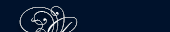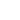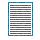НОВОСТИ   БИБЛИОТЕКА   ЭНЦИКЛОПЕДИЯ   КАРТА САЙТА   ССЫЛКИ   О САЙТЕ### SOME METHODS

Addition is best performed when the numbers are written in columns so that units, tens, hundreds, and so forth are written vertically.

For example, the sum of 1,562 and 1,891 is obtained as follows:The addition is performed from right to left. We can easily observe that the addition of 8 to 35 gives the same result when 35 is added to 8. In either of the additions the sum is 43.

Thus - the sum of two or more numbers does not change when the order in which the numbers are added is changed.

Note: Обычно при сложении и вычитании столбиком англичане ставят знаки + и - у последнего столбика.

#### Subtraction of Whole Numbers

Subtraction is an arithmetic operation by means of which one of the addends is obtained, when the sum and another addend are given.

The result is known as the difference of the two given numbers.

The number from which another number is to be subtracted is known as the minuend. The number that is subtracted is known as the subtrahend. Subtraction is an operation opposite to addition. The sign is - (minus).ПОИСК: